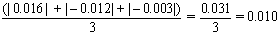The average deviation, is then the sum of the absolute values of the individual deviations, divided by the number of measurements (three). For the data we have been using, the average deviation would be calculated as follows:The mass of the unknown object could then be expressed as 10.127 + 0.010 g.